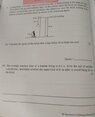# Finding speed of a falling brick using distance and the acceleration of gravity

• vytrx
In summary, the SUVAT equations are necessary for studying physics and understanding the relationship between speed, acceleration, and distance. In this conversation, the question about the falling brick confused the two concepts and resulted in an incorrect answer. The correct approach is to use the SUVAT equations, which can be learned from external resources. f

#### vytrx

Homework Statement
A supervisor 1.8m tall visits a construction site a brick resting at the edge of a roof 50m above the ground suddenly falls at the instant when the brick has fallen 30m without encountering any air resistance, the supervisor sees the brick coming down directly towards him from above as shown in the following figure. (attached) Calculate the speed of the brick after it has falled 30 m from the roof
Relevant Equations
Speed=distance/time
acceleration of gravity is 10m/s^2
Since the brick has fallen 30m and the acceleration of gravity is 10m/s^2 the brick would have fallen 3 seconds speed of brick would then be 30/3 leaving the answer at 10m/s?

I am new to physics and this question has left me stumped

#### Attachments

•1651731802651.jpg
21.5 KB · Views: 49
Relevant Equations:: Speed=distance/time
That's only true for constant speed. Or, in simple cases, for calculating the average speed.

Do you know the SUVAT equations?

Since the brick has fallen 30m and the acceleration of gravity is 10m/s^2 the brick would have fallen 3 seconds
You may be confusing speed and acceleration here.
speed of brick would then be 30/3 leaving the answer at 10m/s?
This is not correct.That's only true for constant speed. Or, in simple cases, for calculating the average speed.

Do you know the SUVAT equations?

You may be confusing speed and acceleration here.

This is not correct.No I'm not familiar with the SUVAT equations, yes I may be confusing the two would you care to explain how this question is done? I've asked one of my friends that said that the answer was 24.5 but I'm not entirely sure how to get there

No I'm not familiar with the SUVAT equations, yes I may be confusing the two would you care to explain how this question is done? I've asked one of my friends that said that the answer was 24.5 but I'm not entirely sure how to get there
The SUVAT equations are the starting point for a study of physics, so you'll need to learn about them. Try this to get you started.

https://www.ncl.ac.uk/webtemplate/a...mechanics/kinematics/equations-of-motion.html

•Grelbr42
•Lnewqban
•Grelbr42 and Lnewqban